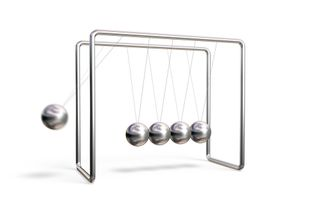# How Does Newton's Cradle Work?Newton's Cradle demonstrates some simple laws of physics.

Whether you know it as Newton's Cradle or the Executive Ball Clicker, chances are you've seen the educational desk toy that seems to defy explanation. The device consists of a row of five metal balls — positioned to just barely touch one another — suspended from a frame by thin wires.

When a ball on one end of the cradle is pulled away from the others and then released, it strikes the next ball in the cradle, which remains motionless. But the ball on the opposite end of the row is thrown into the air, then swings back to strike the other balls, starting the chain reaction again in reverse.

How does Newton's Cradle work? The device can be explained with some of the fundamental principles of physics and mechanics (as theorized by Sir Isaac Newton, René Descartes and others).

Newton's Cradle aptly demonstrates the principle of the conservation of momentum (mass times speed). This principle states that when two objects collide, the total momentum of the objects before the collision is equal to the total momentum of the objects after the collision.

In other words, when the first ball of Newton's Cradle collides with the second, the first ball stops, but its momentum isn't lost, just transferred to the second ball, then the third, then the fourth, until it reaches the very last ball. You witness this conservation of momentum as the last ball swings into the air with nearly the same momentum as the first ball.  Thus, if two balls are lifted into the air on one end of the device and released, then two balls on the opposite end will swing in response.

This continuous clicking of balls is also proof of Newton's law of the conservation of energy, which states that energy can't be created or destroyed but that it can change forms. Newton's Cradle demonstrates this last part of the law quite well, as it converts the potential energy of one ball into kinetic energy that is transferred down the line of balls and ultimately results in the upward swinging of the last ball.